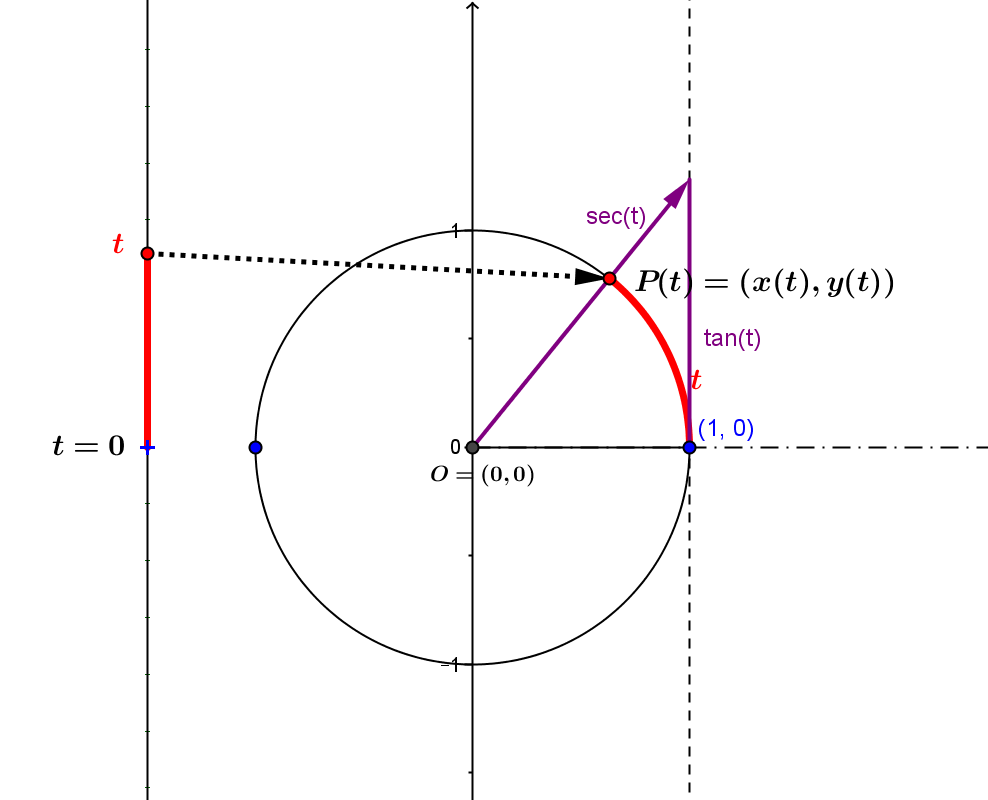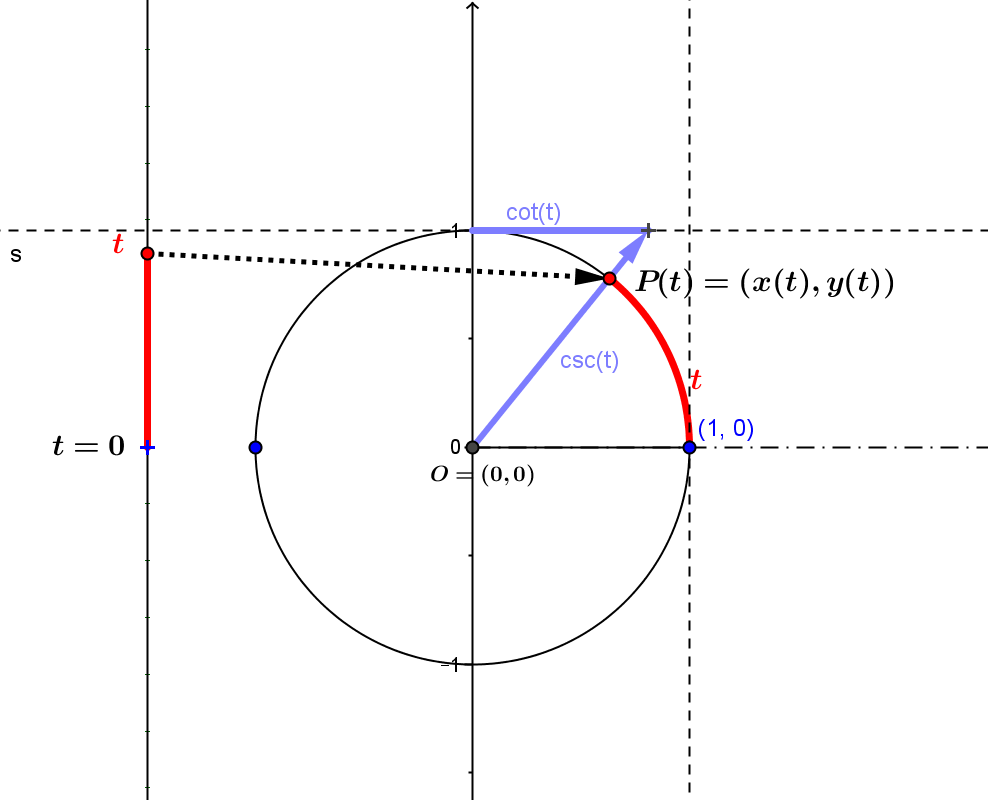The secant, cosecant, and cotangent functions:
(i) Using The Reciprocal Function.
(ii) Using The Unit Circle.

(i) Whether using triangles or the unit circle, the multiplicative inverses of the sine and cosine and tangent functions can define the cosecant, secant, and cotangent functions at all cases except that would lead to division by zero.
Thus these other three trigonometric functions can be thought of as the composition of the cosine, sine and tangent functions with the function $r(x) = 1/x, x\ne 0$.

$\sec(t) = \frac 1{\cos(t)} = r(\cos(t)), t \ne (2k+1)\pi/2, k \in \mathbb Z$;
$\csc(t) = \frac 1{\sin(t)} = r(\sin(t)), t \ne k\pi, k \in \mathbb Z$;  and
$\cot(t) = \frac 1{\tan(t)} = r(\tan(t)), t \ne k\pi, k \in \mathbb Z$.

This can be visualized with mapping diagram  compositions.

(ii) The unit circle approach to the trigonometric functions that considers central angles in a unit circle centered at the point $O=(0,0)$ in a cartesian coordinate plane provides another way to visualize the cosecant, secant, and cotangent functions.
Start with the central angle $t$ that determines the point, $P(t)=(x(t), y(t)$ on the unit circle. Using the lines $x =1$ and $y=1$ and the ray $OP(t)$ gives a sensible way to visualize the values of the other trigonometric functions, the secant, cosecant, tangent and cotangent as lengths of line segments.$\tan(t)$ and $\sec(t)$ $\cot(t)$ and $\csc(t)$

Details: The Unit Circle and The Other Trigonometric Functions